# DAV Class 5 Maths Chapter 4 Worksheet 8 Solutions

The DAV Class 5 Maths Book Solutions Pdf and DAV Class 5 Maths Chapter 4 Worksheet 8 Solutions of Fractional Numbers offer comprehensive answers to textbook questions.

## DAV Class 5 Maths Ch 4 Worksheet 8 Solutions

Question 1.
Fill in the blanks.
(a)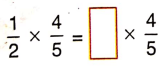Solution: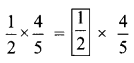(b)Solution:(c)Solution: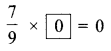(d)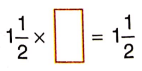Solution: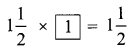(e)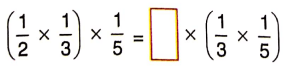Solution:(f)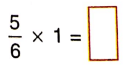Solution:(g)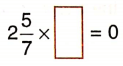Solution: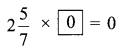(h)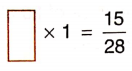Solution:(i)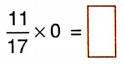Solution: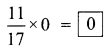(j)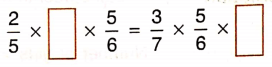Solution: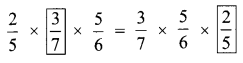DAV Class 5 Maths Chapter 4 Worksheet 8 Notes

I. If two fractional numbers are multiplied in either order, the product remains the same.
E.g., Multiply two fractional numbers $$\frac{1}{5} \times \frac{2}{7}$$
$$\frac{1}{5} \times \frac{2}{7}$$
= $$\frac{2 \times 1}{7 \times 5}$$
= $$\frac{2}{35}$$
Let us interchange the places of fractional numbers.
$$\frac{2}{7} \times \frac{1}{5}$$
= $$\frac{2 \times 1}{7 \times 5}$$
= $$\frac{2}{35}$$
We observe that the product in both cases is the same.
$$\frac{1}{5} \times \frac{2}{7}=\frac{2}{7} \times \frac{1}{5}$$

II. Multiplication of a fractional number by 1.
If a fractional number is multiplied by one the product is the fractional number itself.
E.g., Find $$\frac{5}{3}$$ × 1
$$\frac{5}{3}$$ × 1
= $$\frac{5 \times 1}{3}$$
= $$\frac{5}{3}$$

III. Multiplication of a fractional number by 0.
If a fractional number is multiplied by zero, the product is zero.
E.g., $$\frac{3}{11}$$ × 0 = ?
$$\frac{3}{11}$$ × 0
= $$\frac{3 \times 0}{11}$$
= 0IV. Multiplication of three fractional numbers.
If three fractional numbers are multiplied in any order, the product remains the same.
E.g., What is product of $$\frac{1}{3} \times \frac{1}{5} \times \frac{5}{4}$$ when grouping are changed?
$$\left(\frac{1}{3} \times \frac{1}{5}\right) \times \frac{5}{4}$$ = $$\frac{1}{15} \times \frac{5}{4}$$ = $$\frac{1}{12}$$
Again when grouping is changed.
$$\frac{1}{3} \times\left(\frac{1}{5} \times \frac{5}{4}\right)$$ = $$\frac{1}{3} \times \frac{5}{20}$$ = $$\frac{1}{12}$$